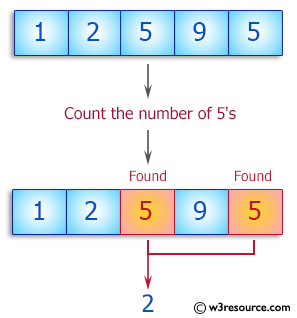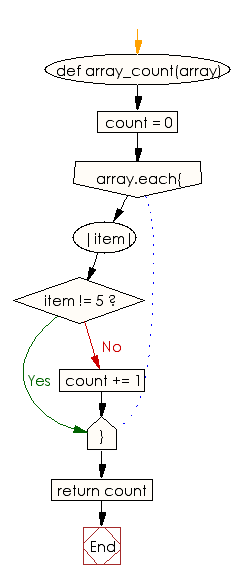﻿ Ruby Basic exercises: Count the number of 5's in a given array - w3resource# Ruby Basic Exercises: Count the number of 5's in a given array

## Ruby Basic: Exercise-41 with Solution

Write a Ruby program to count the number of 5's in a given array.Ruby Code:

``````def array_count(array)
count = 0
array.each{|item| count += 1 unless item != 5}
return count
end

print array_count([1, 2, 9]),"\n"
print array_count([1, 2, 5, 9]),"\n"
print array_count([1, 2, 5, 9, 5])
``````

Output:

```0
1
2
```

Flowchart:Ruby Code Editor: